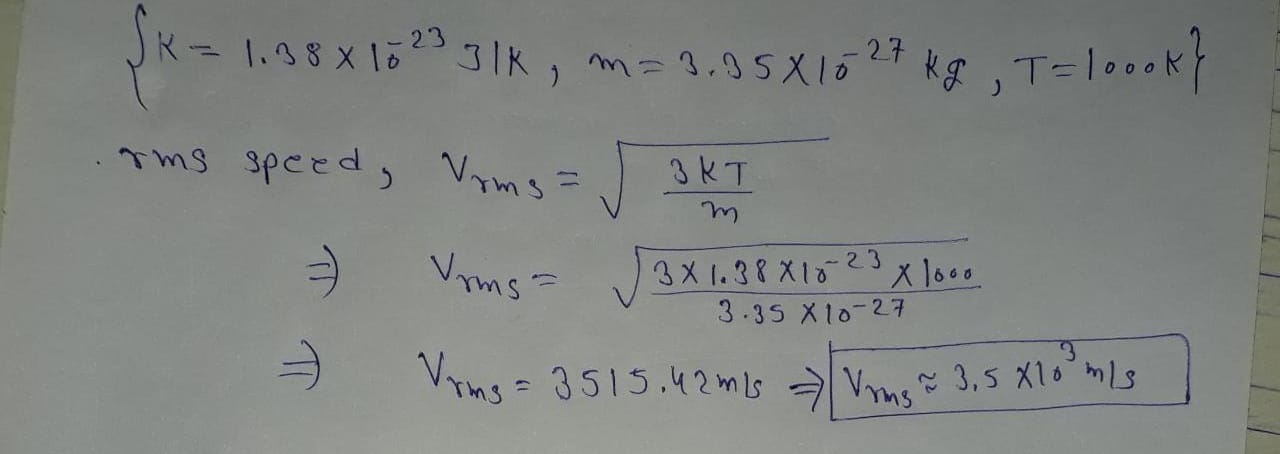In: Physics

# 14. Boltzman's constant is equal to 1.38 x 10-23 J/ K. Hydrogen gas molecules have a...

14. Boltzman's constant is equal to 1.38 x 10-23 J/ K. Hydrogen gas molecules have a mass of 3.35 x 10-27 kg. At 1000 K, what would be the rms speed of these H2 molecules?

a. less than 1 m/s

b. 3.5 x 103 m/s

c. 1.2 x 107 m/s

d. more than 109 m/s

e. none of these

## Solutions

##### Expert Solution## Related Solutions

##### Determine the number of moles in 4.21 x 10^23 molecules of CaCl2
Determine the number of moles in 4.21 x 10^23 molecules of CaCl2
##### An ideal gas is expanding adiabatically from 314.3 K to 243.3 K, creating 3280 J of...
An ideal gas is expanding adiabatically from 314.3 K to 243.3 K, creating 3280 J of work. What type of gas is it?
##### A mass of m = 1 Kg of an ideal gas (gas constant R= 278 J/KgK)...
A mass of m = 1 Kg of an ideal gas (gas constant R= 278 J/KgK) undergoes two polytropic processes. During the first process temperature increases from 27 0C to 237 0C and volume decreases from 1 m3 to 0.3 m3. During the second process temperature increases to 473 0C and volume is constant. The isentropic exponent of the gas is 1.4. Determine (a) Polytropic exponents (b) missing properties of the gas (c) heat and work of the first process...
##### 10.   The initial and final entropy of a system are 42.9 J/mole*K and 267.2 J/mole*K, respectively....
10.   The initial and final entropy of a system are 42.9 J/mole*K and 267.2 J/mole*K, respectively. At a temperature of 86.3oC, the enthalpy associated with this process was +68.6 KJ/mole. (a)        Calculate the number of configurations that the system can assume finally. (b)       Calculate the change of entropy for the system, in J/mole*K. Also, state whether the system became more or less disorderly. (c)        Calculate the Gibb’s free energy for the process, in KJ/mole (d)       State whether the process above...
##### The equilibrium constant, K, for the following reaction is 2.3 x 10-4 at 300 °C: N2...
The equilibrium constant, K, for the following reaction is 2.3 x 10-4 at 300 °C: N2 (g) + C2H2 (g) ⇌ 2 HCN (g). Calculate the equilibrium concentration of HCN when 0.555 moles of N2 and 0.555 moles of C2H2 are introduced into a 0.500 L vessel at 300 °C.
##### The Henry's law constant for nitrogen gas is 3.91 x 10-2 molal/atm at 20°C. How many...
The Henry's law constant for nitrogen gas is 3.91 x 10-2 molal/atm at 20°C. How many moles of nitrogen gas would be dissolved in a 22.0L aquarium at 20°C, assuming an atmospheric pressure of 1.00 atm, and that the partial pressure of nitrogen gas in air is 0.77 atm? Assume the aquarium water has a density of 1.00 kg/L.
##### FIreclay brick (ρ=2050 kg/m3, cp=960 J/kg-K, k=1.1 W/m-K) with dimensions 0.06 m x 0.09 m x...
FIreclay brick (ρ=2050 kg/m3, cp=960 J/kg-K, k=1.1 W/m-K) with dimensions 0.06 m x 0.09 m x 0.20 m is removed from a kiln at 1600K and cooled in air at 40C with h = 30 W/m2-K. What is the temperature at the corner of the brick after 50 minutes of cooling? Give your answer in degrees C.
##### How can a hydrogen bond be weaker than a covalent bond if molecules that have hydrogens...
How can a hydrogen bond be weaker than a covalent bond if molecules that have hydrogens bond have higher boiling point that the ones with covalent bonds?
##### The equilibrium constant, K, for the following reaction is 1.87×10-2 at 511 K. PCl5(g) <--------->>>PCl3(g) +...
The equilibrium constant, K, for the following reaction is 1.87×10-2 at 511 K. PCl5(g) <--------->>>PCl3(g) + Cl2(g) An equilibrium mixture of the three gases in a 13.1 L container at 511 K contains 0.209 M PCl5,   6.24×10-2 M PCl3 and 6.24×10-2 M Cl2. What will be the concentrations of the three gases once equilibrium has been reestablished, if the equilibrium mixture is compressed at constant temperature to a volume of 7.01 L? [PCl5] = M [PCl3] = M [Cl2] =...
##### Un-ionized hydrogen cyanide (HCN, Ka = 4.8 x 10-10) is toxic to fish. If the toxic...
Un-ionized hydrogen cyanide (HCN, Ka = 4.8 x 10-10) is toxic to fish. If the toxic level for un-ionized hydrogen cyanide (HCN) for a certain fish species of concern is 10-6 mol/L (i.e, the equilibrium concentration for HCN), at what pH does the HCN reach toxic levels?